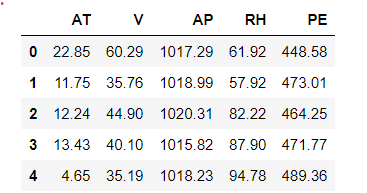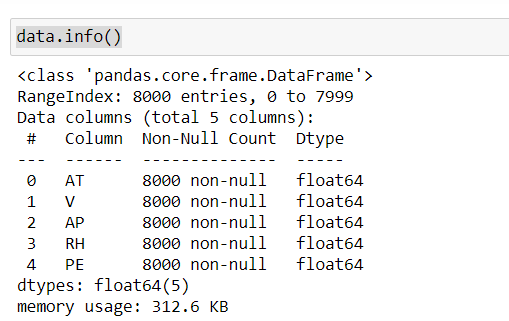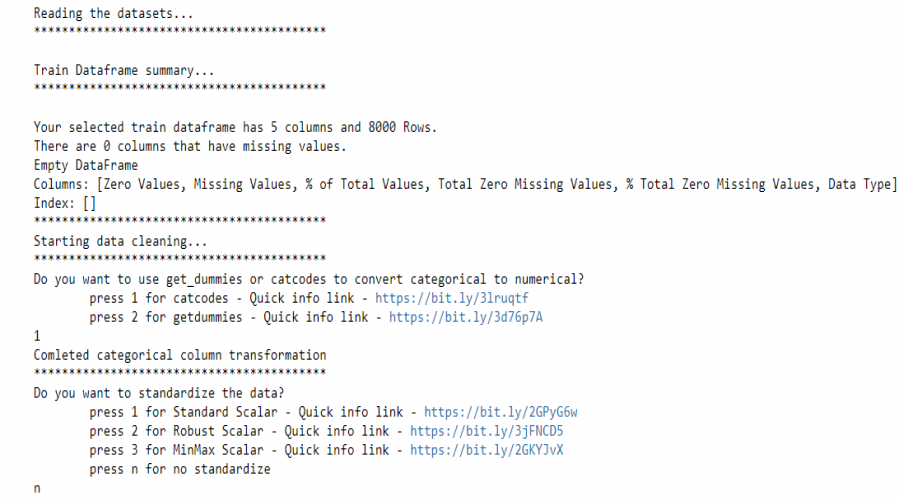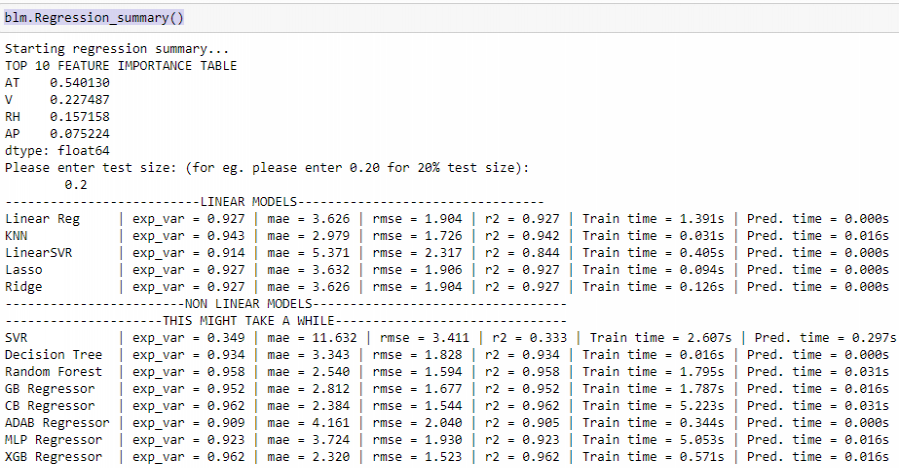# Pywedge package for Machine Learning problems

• Last Updated : 09 Mar, 2021

When people start to learn machine learning and data science, one fact/observation they will always hear is that fitting of machine learning models to a dataset is easy but preparing the dataset for the task is not. While solving ML problems we are often required to go through a series of steps before we can actually find the best ML algorithm that fits accurately onto our dataset. Few major steps can be named as:

1. Collection of data: It can be collected from various sources either from real-life data or can be made manually.
2. Dataset preprocessing: After collecting the raw data, we need to convert it into a meaningful form, so that it can be well interpreted by the algorithms. It also involves a series of steps such as- understanding the data using exploratory data analysis, removes the missing values in the dataset (by imputation methods/manually).
3. Feature engineering: In feature engineering, we implement process such as converting categorical features into numerical features, standardization, normalization, feature selection using different methods such as chi-square test, using extra tree classifier.
4. Handling imbalance in dataset: Sometimes the dataset we collect is in highly imbalanced state. Fitting any model to this type of dataset can give us inaccurate results because the model always has a bias towards the frequently occurring data inside the dataset.
5. Making baseline models: In this we fit different ML algorithms on our data and try to figure out which model gives us more accurate result.
6. Hyperparametertuning: After we select the best model from all the models, we tune the hyperparameters of the model in order to increase accuracy of our model by solving the problem of underfitting/ overfitting.

Thus, we can conclude before getting our desired results, we have to undergo a lot of different steps. Talking in terms of time, around 80% of the time is consumed in data preparation so that model can fit onto it and rest 20% is required for fitting on ML algorithms and making predictions. Thus, it is surely an exhaustive task to carry out all these tasks, but what if we can use some method/function/library so that our this task becomes easy.

### What is Pywedge?

Pywedge is an open-source python package and is pip-installable which is developed by Venkatesh Rengarajan Muthu and it can help us to automate the task of writing code for data preprocessing, data visualization, feature engineering, handling imbalanced data, and making standard baseline models, hyperparameter tuning in a very interactive manner.

Features of Pywedge:

1. It can make 8 different types of interactive charts such as: Scatter plot, Pie Chart, Box plot, Bar plot, Histogram,etc.
2. Data preprocessing using interactive methods such as handling of missing values, converting categorical features into numerical features, standardization, normalization, handling class imbalance, etc.
3. It automatically fits our data onto different ML algorithms and gives us 10 best baseline models.
4. We can also apply hyperparameter tuning on our desired model.

Let’s use this pywedge library to solve a regression problem in which we have to predict the energy generated by a powerplant using the dataset taken from Dockship’s Power Plant Energy Prediction AI Challenge.

## Python3

 `import` `numpy as np``import` `pandas as pd``import` `warnings``warnings.filterwarnings(``"ignore"``)``from` `sklearn.model_selection ``import` `train_test_split``from` `sklearn.metrics ``import` `mean_squared_error`

## Python

 `# Loading testing Data``test_data ``=` `pd.read_csv(``"TEST.csv"``)``# Loading training Data``data ``=` `pd.read_csv(``"TRAIN.csv"``)``# Printing the shape of train dataset``data.shape`

`(8000, 5)`

Now, we will check how our dataset looks like using the head() method and check some of its information in the subsequent step as:

## Python

 `data.head()`We can infer from the above image that our dataset has 5 columns in which the first four columns are our features and last column (PE) is our target column.

## Python

 `data.info()`Using the info() method, we can interpret that our dataset has no missing values and data type of each feature is of type float64.

Using pywedge library:

## Python

 `import` `pywedge as pw``ppd ``=` `pw.Pre_process_data(data, test_data, y``=``'PE'``,c``=``None``,``type``=``"Regression"``)``new_X, new_y, new_test ``=` `ppd.dataframe_clean()`We use pywedge’s Pre_process_data method to load the training data and create a Pre_process_data object, the object has a dataframe_clean method which returns pre-processed data. This method interactively asks for methods to convert categorical features into numerical features and also gives options to choose different standardization techniques to standardize the dataset.

#### Preparing baseline models using pywedge:

Making the modified train and test data and preparing the baseline models-

## Python

 `# Assigning preprocessed data to make train and test data``X_train ``=` `new_X``y_train ``=` `new_y``X_test ``=` `new_test``# calling baseline_model method to prepare all the baseline models``blm ``=` `pw.baseline_model(X_train,y_train)``# printing the regression summary``blm.Regression_summary()`standard baseline models

The baseline_model method creates an object ‘blm’ and Regression_summary() method returns a summary about the implemented models. It gives us the top 10 most important features calculated using AdaBoost regressor and best baseline models. Also, we can check which algorithm takes how much time to train and make predictions. Different metrics using which we evaluate our model is also displayed. However, it does not perform any hyperparameter tuning so the best model can later be fine-tuned to get more accurate results.

Thus, we can notice how quickly we can find out which machine learning model we should use for our problem by just writing a few lines of code.

My Personal Notes arrow_drop_up